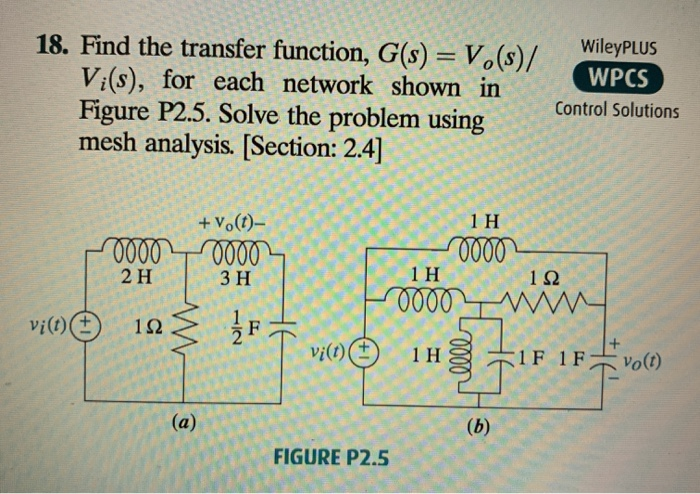### Create an Account

Home / Questions / 18. Find the transfer function, G(s) = V.(s)/ Vi(s), for each network shown in Figure P2.5. Solve th

# 18. Find the transfer function, G(s) = V.(s)/ Vi(s), for each network shown in Figure P2.5. Solve th18. Find the transfer function, G(s) = V.(s)/ Vi(s), for each network shown in Figure P2.5. Solve the problem using mesh analysis. [Section: 2.4] WileyPLUS WPCS Control Solutions Co. + v.(t)- "00002 VO SA vi(n) 21 | 3H in je 11 12 Froon min Hg 1F1Fvo(1) T vict) FIGURE P2.5

Apr 06 2020 View more View LessSubscribe To Get Solution